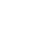Not a developer? Go to MovableType.com

Plugin and Theme Directory

# itex2MML v0.9

By Jacques Distler
Posted February 16, 2003, in Editing.

## Details

For Math equations in entries - compose posts in itex, and converts them to XHTML+MathML, which advanced browsers like Mozilla can render.

$\sin(\theta)\cos(\theta)= \frac{1}{2}\sin(2\theta)$


is an inline equation.

$\int_{-\infty}^{\infty} e^{-x^2} dx = \sqrt{\pi}$


is a display equation.

### Jacques DistlerProfessor of Physics at the University of Texas at Austin. MT-hacker since 2002.

Website: http://golem.ph.utexas.edu/~distler/blog/
• #### Popular Plugins